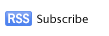graphs of functions

To graph functions, we use coordinate plane. A function has an input which is x, and its corresponding output f(x) which is y. The ordered pair (x,y) is a point, and to graph a function, we connect all the points produced by the function in the xy-plane.Blog Summary Widget